Related Rates | Volume Integration | Polar Calculus | Integration by Parts | Quiz

Volume Integration

In calculus there are two main methods of calculating the volume generated by the revolution of an area about an axis. Either method will work on any given problem. In some problems, it is "easier" to use one over the other.

They are...

 THE DISK METHOD A method that finds the volume of a revolution about an axis by summing cylinders of infinitesmal width about the axis.

and

 THE SHELL METHOD A method that finds the volume of a revolution about an axis by summing the shells of infinitesmal width about the axis.

Don't worry if that didn't make sense to you, it will by the end of this series of pages.

The Disk Method about the x or y axis.

This method comes from the idea of using many disks of radius R and width dx or dy over an interval. Notice that the infinitesmal element is perpendicular to the axis of revolution.

To represent this, we can write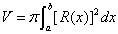for about a horizontal axis, or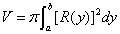for about a vertical axis.

Example 1: Find the volume generated by the rotation of the region bounded by y = (x-4)(x-2) x^2-6x+8 and y = 0 about the x axis.

First we need to find the bounds. In other words, what are the roots? They are 4 and 2.
Using the integral given, we just substitute in the bounds and the function.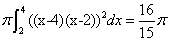Example 2: Find the volume generated by the rotation of the region bounded by x = (y+1)(y-3) and x = 0 about the y axis.
Instead of having the integral with respect to dx, we change the infinitesmal element to dy.
The bounds for this integral are now along the y axis, instead of the x axis.
First we need to find the bounds. In other words, when does x = (y+1)(y-3) intersect x = 0? It intersects when y = -1 or 3.
So, using the integral given, we just substitute in the bounds and the function to get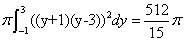.

The Disk Method about an axis that is not x = 0 or y = 0.

In the previous two examples, the problems dealt with a revolution about the x or y axis. What happens when the function is revolved about a different axis such as x = 3 or y = 2?

This changes the part of the integral that we want to square, because it is changing the radius of the disk. It might help to draw a picture to make it clear what is the representative function of the radius.

Example 3: Find the volume generated by the rotation of the region bounded by y = (x-4)(x-2) and y = 0 about y = 3.
Previously we had the radius of the disk just the function. Changing the axis, thus changes the radius. We have to determine this new radius in terms of x's and constants.
To do so, we find the points of intersection between y = 3 and y = (x-4)(x-2). They are x = 1 or x = 5. Since these intersection points are outside the points of intersection between (x-2)(x-4) and 0,
we then have the same bounds, 2 and 4. So, we find the volume that would be generated by this entire volume, and then we want to subtract out that inner volume.
So we do..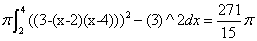Applications of the Disk Method.

The disk method can be used to find more than just the volume of a solid. It can be extended to find the volume of a solid with a hole in it, such as a washer or a donut. To find the volume, we use the disk method twice. Once for the outer volume and once for the inner volume. Subtracting the inner volume from the outer volume leaves the desired volume.

The Shell Method about the x or y axis

The shell method is an extension of the disk method, in that to find the shell, it is the difference between the outer and inner volume. However, the infinitesmal element is no longer perpendicular to the axis of revolution, it is parallel.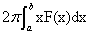for a vertical axis of revolution, where x is the distance to the vertical axis of revolution and F(x) represents the function/height.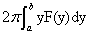for a horizontal axis of revolution, where y is the distance to the horizontal axis of revolution and F(x) represents the function/height.

Example 1: Find the volume generated by the rotation of the region bounded by y = x^(1/2) and y = 0 about the y axis on the interval 0 to 4.
Remembering what separates this from the disk method is that we don't square the radius here, and we include a variable that represents the distance from the axis. Also, the constant is different.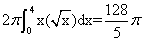a subsidiary of HivePC. Copyright 2005